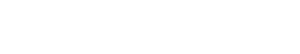# Profit

Profit is a measure of the financial performance of a company. It is typically calculated by subtracting the cost of goods sold from the revenue generated. In addition, profit is a key metric for businesses and is often used to measure the success of a company.

## Basic Types of Profits

There are two types of profit: gross profit and net profit.

• Gross profit is the total revenue minus the cost of goods sold. This is the most basic measure of a company’s profitability. for example, a company that generates \$100 in revenue and has a cost of goods sold of \$80 would have a gross profit of \$20.
• Net profit is the total revenue minus the cost of goods sold and all other expenses. This is the most comprehensive measure of a company’s profitability. For example, a company that generates \$100 in revenue, has a cost of goods sold of \$80, and has \$10 in expenses would have a net profit of \$10.

## Profit Margin

Profit margin is a measure of a company’s profitability. It is calculated by dividing the net profit by the total revenue. For example, a company with a net profit of \$10 and total revenue of \$100 would have a profit margin of 10%.

## Profitability Ratios

Several ratios measure a company’s profitability. The most common are the gross profit margin, net profit margin, and return on assets (ROA).

The gross profit margin measures the percentage of revenue that is left after the cost of goods sold is deducted. For example, a company with a gross profit of \$20 and total revenue of \$100 would have a gross profit margin of 20%.

The net profit margin measures the percentage of revenue that is left after all expenses are deducted. For example, a company with a net profit of \$10 and total revenue of \$100 would have a net profit margin of 10%.

ROA measures the profitability of a company’s assets. It is calculated by dividing the net profit by the total assets. For example, a company with a net profit of \$10 and total assets of \$100 would have a ROA of 10%.Unlock the power of actionable insights with AI-based natural language processing.